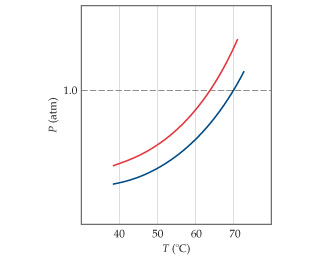# Problem: The following diagram shows the vapor pressure curves of a volatile solvent and a solution of that solvent containing a nonvolatile solute. Which line represents the solution?

91% (50 ratings)
###### Problem Details

The following diagram shows the vapor pressure curves of a volatile solvent and a solution of that solvent containing a nonvolatile solute.Which line represents the solution?

Frequently Asked Questions

What scientific concept do you need to know in order to solve this problem?

Our tutors have indicated that to solve this problem you will need to apply the The Colligative Properties concept. You can view video lessons to learn The Colligative Properties. Or if you need more The Colligative Properties practice, you can also practice The Colligative Properties practice problems.